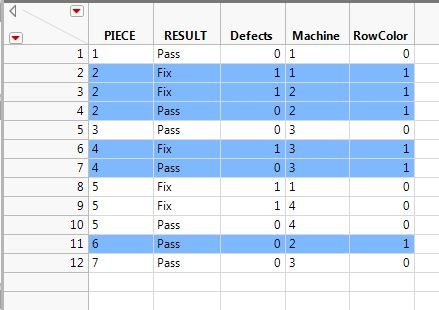Choose Language Hide Translation Bar
Highlighted

## Select Cell Color for Row Based on one Cell's Value

Hello JMP Discussions.  I think this is an easy one but everything I have researched and everything I have tried has failed.

Is it possible to color all cells in a row based on the value found in a column of that row?  I want to select a color that is readable for selected row and leave ones that don't fit the criteria with default color.  If I use Color Rows by Row State the data is not readable using the default color scheme.  I need a table that will highlight the entire row when criteria is met.  Ex.  If Col_1 = 1 then color all of the cells in this row (Col_2, Col_3, Col_4, etc.) a light blue, otherwise, do nothing.

1 ACCEPTED SOLUTION

Accepted Solutions
Highlighted

## Re: Select Cell Color for Row Based on one Cell's Value

You have to color cell by cell.  Try this code:

``````dt = New Table( "test", Add Rows( 6 ),
New Column( "Column 1", Numeric, "Continuous", Format( "Best", 12 ),
Set Values( [0, 1, 1, 0, 1, 0] ) ),
New Column( "Column 2", Character, "Nominal",
Set Values( {"A", "B", "C", "D", "E", "F"} ) ),
New Column( "Column 3", Character, "Nominal",
Set Values( {"G", "H", "I", "J", "K", "L"} ) )
);

// Make the rows light gray
light_gray = rgb color(200, 200, 200);

my_rows = dt << get rows where(:Column 1 == 1);
my_row_list = as list(my_rows);
column_list = dt << get column names(String);
for (i = 1, i <= nitems(column_list), i++,
column(dt, column_list[i]) << color cells(light_gray, my_row_list);
);
``````
7 REPLIES 7
Highlighted

## Re: Select Cell Color for Row Based on one Cell's Value

try this:

``````Names Default To Here( 1 );
dt = Open( "\$SAMPLE_DATA/Big Class.jmp" );
dt << Color by Column( :Age );
Wait( 2 );
dt << Color Rows by Row State;``````

Highlighted

## Re: Select Cell Color for Row Based on one Cell's Value

@ron_horne I've tried that but it gives me red and blue and the blue is nearly impossible to read the text in each row.  I'd prefer a lighter color for one row condition and no color for the other.  I cannot figure out how to do that!  I've tried all manner of options.  Most I've deleted in exasperation so I can't include in my discussion.

Highlighted

## Re: Select Cell Color for Row Based on one Cell's Value

perhaps try setting the colors manualy as such

``````:age << Set Property(

"Value Colors", // assign the value colors

{12 = Red, 13 = Yellow, 14 = Green, 15 = Blue, 16 = Magenta, 17 =

Gray}

);``````

## Re: Select Cell Color for Row Based on one Cell's Value

@ron_horne, Thanks for trying to help me out. However, I feel that I am not being clear.  What I'm looking for is the following based on the value in :RowColor

I want the whole row for RowColor = 1 to be light blue/Color(59)Highlighted

## Re: Select Cell Color for Row Based on one Cell's Value

``````dt << Select Rows( dt << Get Rows Where( :RowColor == 1 ) );

Rws = dt << Get Selected Rows;
Rws = AsList(Rws);
dt << Color Cells( Color(59), Rws );``````

Just tried this and the rows are selected but I cannot change the color of any cells using this code.  Trying to discover what step I'm missing as this is the logic I would follow to color the cells manually.

Highlighted

## Re: Select Cell Color for Row Based on one Cell's Value

You have to color cell by cell.  Try this code:

``````dt = New Table( "test", Add Rows( 6 ),
New Column( "Column 1", Numeric, "Continuous", Format( "Best", 12 ),
Set Values( [0, 1, 1, 0, 1, 0] ) ),
New Column( "Column 2", Character, "Nominal",
Set Values( {"A", "B", "C", "D", "E", "F"} ) ),
New Column( "Column 3", Character, "Nominal",
Set Values( {"G", "H", "I", "J", "K", "L"} ) )
);

// Make the rows light gray
light_gray = rgb color(200, 200, 200);

my_rows = dt << get rows where(:Column 1 == 1);
my_row_list = as list(my_rows);
column_list = dt << get column names(String);
for (i = 1, i <= nitems(column_list), i++,
column(dt, column_list[i]) << color cells(light_gray, my_row_list);
);
``````
Highlighted

## Re: Select Cell Color for Row Based on one Cell's Value

Thank you @pmroz!!! That is what I had missed!  All the other "For" statements I attempted previously didn't get me there.  Now I see where I went wrong.

THANK YOU, THANK YOU!

Article Labels

There are no labels assigned to this post.